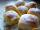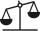# Warehouses

To four warehouses is going cement in 25 kg bags. To first one third, to second quarter of the total. The third store got two thirds of the rest, and the last 310 tons came to fourth. How many cement is in all warehouses and how much got every one?

Result

t (total) =  2232 ton
m1 =  744 ton
m2 =  558 ton
m3 =  620 ton
m4 =  310 ton

#### Solution:Leave us a comment of example and its solution (i.e. if it is still somewhat unclear...):Be the first to comment!#### To solve this example are needed these knowledge from mathematics:

Need help calculate sum, simplify or multiply fractions? Try our fraction calculator. Do you have a linear equation or system of equations and looking for its solution? Or do you have quadratic equation?

## Next similar examples:

1. CakesOn the bowl were a few cakes. Jane ate one-third of them, Dana ate a quarter of those cakes that remained. a) What part (of the original number of cakes) Dana ate? b) At least how many cakes could be (initially) on thebowl?
2. Jam cakesMom baked a third of plum jam cakes, one third cheesecakes and 18 poppy. How many cakes she had bake?
3. BrickIsosceles scale has on one side all brick and second weight 1 kg and 1/4 of brick. The balance is in equilibrium. What is the weight of a brick?
4. BenchesThe park has 64 benches. Occupied are by 18 more than empty. How many benches are occupied and empty ?
5. Mixed2improperWrite the mixed number as an improper fraction. 166 2/3
6. ZdeněkZdeněk picked up 15 l of water from a 100-liter full-water barrel. Write a fraction of what part of Zdeněk's water he picked.
7. Forest nurseryIn the forest nursery after winter, they found that 1/10 stems died out of them. For them, they land 193 new spruces. How many spruces are in the forest nursery?Mom bake cookies. Rolo took 2/9 of all cookies, Michal 3/9. How many cookies ate Rolo if Michal had 9.
9. Equation 20In given equation: 8/9-4/5=2/9+x, find x
10. If-then equationIf 5x - 17 = -x + 7, then x =
11. Lengths of the poolMiguel swam 6 lengths of the pool. Mat swam 3 times as far as Miguel. Lionel swam 1/3 as far as Miguel. How many lengths did mat swim?
12. Cakes1/3 poppy cake, 1/3 apple, 15 pieces of cheese. How many are totally cakes?Added together and write as decimal number: LXVII + MLXIVWrite the fraction 3/22 as a decimal.If 3t-7=5t, then 6t=Write as a fraction and a decimal. One and two plus three and five hundredths3 children had to divide 4 pounds is candy. How much candy did each child get?